Next: Applications of Bernoulli's equation Up: Steady flows of incompressible Previous: Ideal fluids and Euler's

## Bernoulli's equation

Since we are now concentrating on steady flows,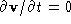. Taking the dot product ofwith Eq. (2.22), and remembering that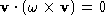, we have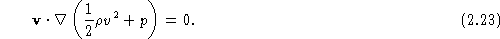If we now consider displacements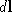along a streamline, sinceis tangent to the streamline Eq. (2.23) becomes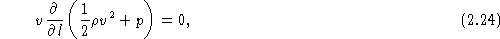or,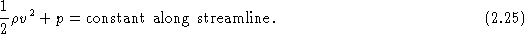This is the famous Bernoulli's equation  for steady flow in a nonviscous fluid. If, in addition, the fluid is irrotational so that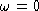, then we have a stronger form of Bernoulli's equation: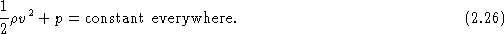We've derived Bernoulli's equation in a somewhat mathematical fashion, but keep in mind that it is simply a statement of conservation of energy in the fluid. The term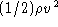is the kinetic energy density of the fluid, and the pressure p can be thought of as a type of potential energy (per unit volume).

Vittorio Celli
Wed Sep 10 01:02:02 EDT 1997